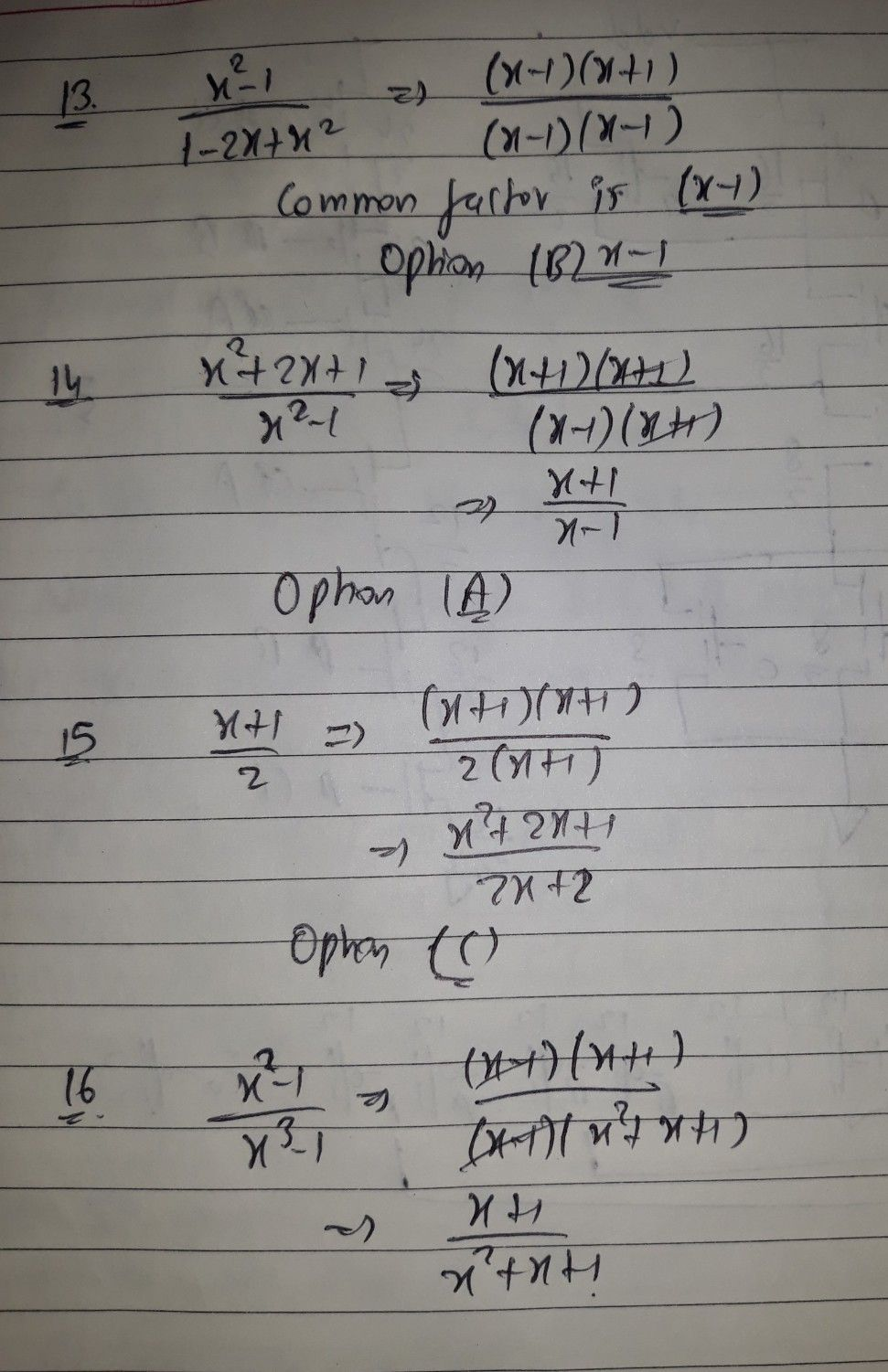Symbol
Problem$-13$ In the rational algebraic expression $\dfrac {x^{2}-1} {1-2x+x^{2}}$ what factor is common to both numerator and denominator? $A.x+1$ B. $x-1$ $C.x^{2-1}$ D. $x^{2}+1$ 14. Which of the following is the simplest form of $\dfrac {x^{2}+2x+1} {x^{2}-1}7$ A. Xx-+1 1 B. xx+-1 1 C. x1-+x 1 D. $\dfrac {1-x} {x+1}$ 15. Which of the following rational expression has $\dfrac {x+1} {2}$ as simplest form? $x^{2}-1$ $-$ C. $\dfrac {x^{2}+2x+1} {2x+2}$ D. $\dfrac {\left(x+1\right)\left(x+2\right)} {2x+2}$ $A.\dfrac {2x+1} {4}$ B. $2x+2$ 5. Which of the following is the simplest form of $\dfrac {x^{2}-1} {x^{3}-1}7$ $\dfrac {1} {x-1}$ X-1 $C.\dfrac {x+1} {x^{2}+x+1}$ D. $\dfrac {x+1} {x^{2}-x+1}$
7th-9th grade
Algebra
Search count: 118
SolutionQanda teacher - anudeepk